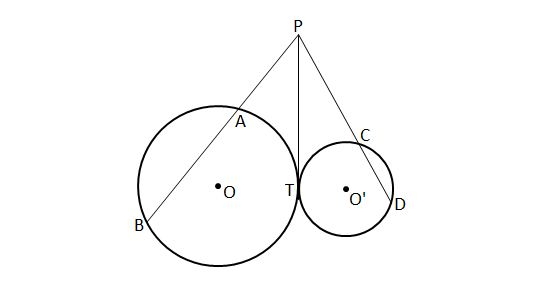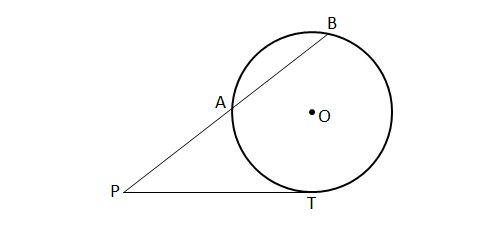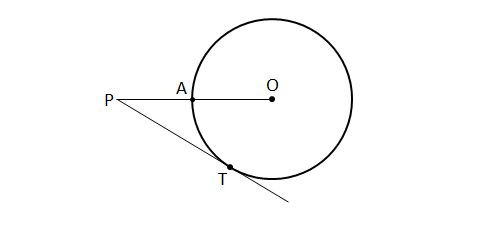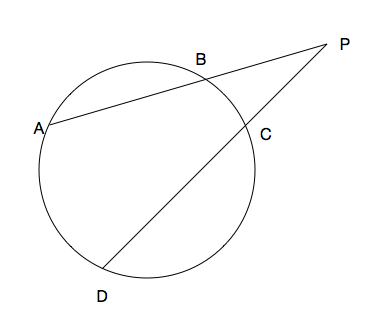Geometry

# Tangent-Secant$\overline{PT}$ is tangent to both circle $O$ and circle $O'$ at the same point $T,$ while $\overline{PAB}$ and $\overline{PCD}$ are secant lines to circle $O$ and circle $O',$ respectively. Given the following three lengths: $\lvert{\overline{AB}}\rvert=25, \lvert{\overline{PC}}\rvert=6, \lvert{\overline{CD}}\rvert=8,$ what is $\lvert\overline{PA}\rvert?$

Note: The above diagram is not drawn to scale.$\overline{PT}$ is tangent to circle $O$ at point $T,$ while $\overline{PAB}$ is a secant line to the circle. If $\lvert\overline{PA}\rvert=16 \text{ and } \lvert\overline{AB}\rvert=6,$ where $\lvert\overline{PA}\rvert$ denotes the length of $\overline{PA},$ what is $\lvert\overline{PT}\rvert ?$

Note: The above diagram is not drawn to scale.$\overline{PT}$ is tangent to circle $O$ at point $T,$ and we are given the following two lengths: $\lvert{\overline{PT}}\rvert=22 \text{ and } \lvert{\overline{PA}}\rvert=18.$ What is the radius of circle $O ?$

Note: The above diagram is not drawn to scale.In the above figure, $A, B$ and $T$ are three points lying on a circle. If $\overline{PT}$ is a tangent line of the circle and $\lvert\overline{AB}\rvert=7 \text{ and }\lvert\overline{PT}\rvert=12,$ where $\lvert\overline{AB}\rvert$ denotes the length of $\overline{AB},$ what is $\lvert\overline{PA}\rvert ?$

Note: The above diagram is not drawn to scale.From a point $P$ outside of the circle, 2 lines are drawn which intersect the circle at $A$ and $B$, $C$ and $D$ respectively. If $|PA| = 16$, $|PB| = 7$ and $|PC| = 4$, what is the length $|PD|$?

×